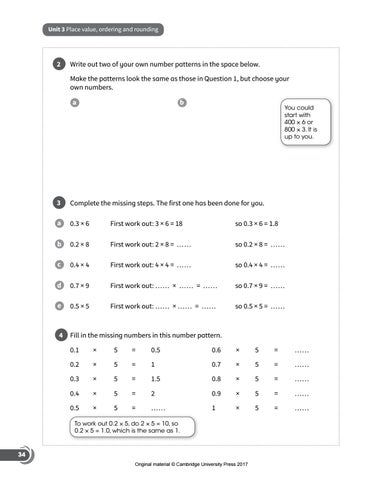# Preview Cambridge Checkpoint Mathematics Skills Builder 7Unit 3 Place value, ordering and rounding

2

Write out two of your own number patterns in the space below. Make the patterns look the same as those in Question 1, but choose your own numbers. a

b

You could start with 400 × 6 or 800 × 3. It is up to you.

3

Complete the missing steps. The first one has been done for you.

a

0.3 × 6

First work out: 3 × 6 = 18

so 0.3 × 6 = 1.8

b

0.2 × 8

First work out: 2 × 8 = ……

so 0.2 × 8 = ……

c

0.4 × 4

First work out: 4 × 4 = ……

so 0.4 × 4 = ……

d

0.7 × 9

First work out: …… × …… = ……

so 0.7 × 9 = ……

e

0.5 × 5

First work out: …… × …… = ……

so 0.5 × 5 = ……

4

Fill in the missing numbers in this number pattern. 0.1

×

5

=

0.5

0.6

×

5

=

……

0.2

×

5

=

1

0.7

×

5

=

……

0.3

×

5

=

1.5

0.8

×

5

=

……

0.4

×

5

=

2

0.9

×

5

=

……

0.5

×

5

=

……

1

×

5

=

……

To work out 0.2 × 5, do 2 × 5 = 10, so 0.2 × 5 = 1.0, which is the same as 1.

34 Original material © Cambridge University Press 2017